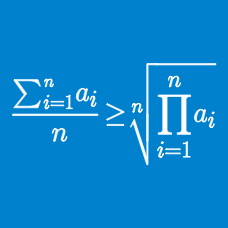Algebra

# Classical Inequality Statements: Level 2 Challenges

$\dfrac{a}{b} + \dfrac{b}{c} + \dfrac{c}{d} + \dfrac{d}{a}$

If $$a, b, c$$ and $$d$$ are any four positive real numbers, then find the minimum value of the expression above.

Let $$x$$ be a positive real number. Find the minimum value of $8x^{5}+10x^{-4}.$

Positive reals $$a,b,c$$ satisfy $$abc=1$$. Find the minimum value of

$\dfrac{1+ab}{1+a}+\dfrac{1+bc}{1+b}+\dfrac{1+ca}{1+c}.$

Enter your answer correct to 3 decimal places.

For $$a,b,c>0$$ and $$a+b+c=6$$. Find the minimum value of

$\large \left (a+\frac{1}{b} \right )^{2}+ \left (b+\frac{1}{c} \right )^{2}+\left (c+\frac{1}{a} \right )^{2}$

Find the minimum value of $$\sqrt{a^2+b^2}$$ when $$3a+4b=15$$.

×

Problem Loading...

Note Loading...

Set Loading...